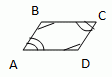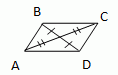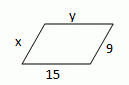Email us to get an instant 20% discount on highly effective K-12 Math & English kwizNET Programs!

#### Online Quiz (WorksheetABCD)

Questions Per Quiz = 2 4 6 8 10

### Geometry3.6 ParallelogramProperties of a Parallelogram: 1. In a parallelogram any two opposite sides are equal. 2. In a parallelogram any two opposite angles are equal. 3. In a parallelogram the diagonals bisect each other. 4. In a parallelogram, each diagonal divides it into two congruent triangles. 5. In a parallelogram, any two adjacent angles have their sum equal to 180�, that is, they are supplementary. THEOREMS: 1. A quadrilateral is a parallelogram, then its opposite sides are congruent;If ABCD is a parallelogram, then AB @ CD and BC @ AD 2. A quadrilateral is a parallelogram, then its opposite angles are congruent;If ABCD is a parallelogram, then �A @ �C and �B @ �D. 3. A quadrilateral is a parallelogram, then its consecutive angles are supplementary;�x + �y = 1800 4. A quadrilateral is a parallelogram, then its diagonals bisect each other.5. One pair of opposite sides are equal and parallel; Special types of parallelograms: rhombus, rectangle, square A quadrilateral is a rhombus if and only if it has four congruent sides. A quadrilateral is a rectangle if and only if it has four right angles. A quadrilateral is a square if and only if it is a rhombus and rectangle. A parallelogram is a rhombus, if and only if its diagonals are perpendicular. A parallelogram is a rhombus, if and only if each diagonal bisects a pair of opposite angles. A parallelogram is a rectangle, if and only if its diagonals are congruent. Example: In a parallelogram ABCD �A = 60�. Find the remaining angles. Solution: We know that the opposite angles of a parallelogram are equal. So � A = � C = 60�. Two adjacent angles of a parallelogram have their sum equal to 180�. Now both �B and �D are adjacent to �A. \ �B = �D = 180� - 60� = 120�. \ The remaining angles are 100�, 80� and 100�. Directions: Draw a parallelogram and answer the following questions. 1) Parallelogram of lengths 5, 12, 5, x+4 cm. Find the value of x. 2) Parallelogram of angles 600, 1200,600, 2x0. Find the value of x. 3) Parallelogram with supplementary angles of 2x0, 4x0. Find the value of x.
 Q 1: Find the value of m in the figure.5612 Q 2: In a parallelogram ABCD, angle A = 85 degrees. Find the remaining angles.95, 85 and 95 degrees Respectively110, 70 and 110 degrees Respectively60, 120 and 60 degrees Respectively100, 80 and 100 degrees Respectively Q 3: Find the value of x in the figure.453050 Q 4: In a parallelogram ABCD, angle A = 120 degrees. Find the remaining angles.110, 70 and 110 degrees Respectively100, 80 and 100 degrees Respectively95, 85 and 95 degrees Respectively60, 120 and 60 degrees Respectively Q 5: Find the value of x in the figure.Answer: Q 6: In a parallelogram ABCD, angle A = 110 degrees. Find the remaining angles.100, 80 and 100 degrees Respectively70, 110 and 70 Respectively110, 70 and 110 degrees Respectively60, 120 and 60 degrees Respectively Q 7: In a parallelogram ABCD, angle A = 70 degrees. Find the remaining angles.95, 85 and 95 degrees Respectively60, 120 and 60 degrees Respectively100, 80 and 100 degrees Respectively110, 70 and 110 degrees Respectively Q 8: Find the value of x and y in the figure.9, 1515, 159, 915, 9 Question 9: This question is available to subscribers only! Question 10: This question is available to subscribers only!